JEE  >  Test: Problems on Integration- 1

# Test: Problems on Integration- 1

Test Description

## 5 Questions MCQ Test Mathematics (Maths) Class 12 | Test: Problems on Integration- 1

Test: Problems on Integration- 1 for JEE 2022 is part of Mathematics (Maths) Class 12 preparation. The Test: Problems on Integration- 1 questions and answers have been prepared according to the JEE exam syllabus.The Test: Problems on Integration- 1 MCQs are made for JEE 2022 Exam. Find important definitions, questions, notes, meanings, examples, exercises, MCQs and online tests for Test: Problems on Integration- 1 below.
Solutions of Test: Problems on Integration- 1 questions in English are available as part of our Mathematics (Maths) Class 12 for JEE & Test: Problems on Integration- 1 solutions in Hindi for Mathematics (Maths) Class 12 course. Download more important topics, notes, lectures and mock test series for JEE Exam by signing up for free. Attempt Test: Problems on Integration- 1 | 5 questions in 10 minutes | Mock test for JEE preparation | Free important questions MCQ to study Mathematics (Maths) Class 12 for JEE Exam | Download free PDF with solutions
 1 Crore+ students have signed up on EduRev. Have you?
Test: Problems on Integration- 1 - Question 1

###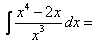Detailed Solution for Test: Problems on Integration- 1 - Question 1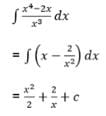Test: Problems on Integration- 1 - Question 2

###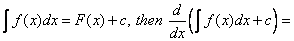Detailed Solution for Test: Problems on Integration- 1 - Question 2

∫f(x) dx = f(x) + c

∫f(x)dx + c = f(x) + c + cx

d/dx(f(x) + k + cx) {As we know that k = c' + c}

f(x) + 0 + c

f(x) + c

Test: Problems on Integration- 1 - Question 3

###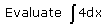​

Detailed Solution for Test: Problems on Integration- 1 - Question 3

Integration of 4dx will be  4x + c

Test: Problems on Integration- 1 - Question 4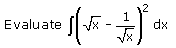Test: Problems on Integration- 1 - Question 5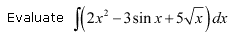## Mathematics (Maths) Class 12

209 videos|209 docs|139 tests
 Use Code STAYHOME200 and get INR 200 additional OFF Use Coupon Code
Information about Test: Problems on Integration- 1 Page
In this test you can find the Exam questions for Test: Problems on Integration- 1 solved & explained in the simplest way possible. Besides giving Questions and answers for Test: Problems on Integration- 1, EduRev gives you an ample number of Online tests for practice

## Mathematics (Maths) Class 12

209 videos|209 docs|139 tests